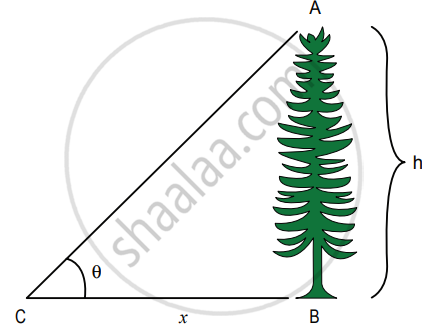Tamil Nadu Board of Secondary EducationHSC Science Class 11th

# Write a note on the triangulation method and radar method to measure larger distances. - Physics

Write a note on the triangulation method and radar method to measure larger distances.

#### Solution

Triangulation method for the height of an accessible object;

Let AB = h be the height of the tree or tower to be measured. Let C be the point of observation at distance x from B. Place a range finder at C and measure the angle of elevation, ACB =
θ as shown in the figure.

From right-angled triangle ABC,Triangulation method

tan θ = "AB"/"BC" = h/x

(or) height h = x tan θ

Knowing the distance x, the height h can be determined.

The word RADAR stands for radio detection and ranging. Radar can be used to measure accurately the distance of a nearby planet such as Mars. In this method, radio waves are sent from transmitters which, after reflection from the planet, are detected by the receiver. By measuring, the time interval (t) between the instants the radio waves are sent and received, the distance of the planet can be determined as to where v is the speed of the radio wave. As the time is taken (t) is for the distance covered during the forward and backward path of the radio waves, it is divided by 2 to get the actual distance of the object. This method can also be used to determine the height, at which an airplane flies from the ground.

d = (v xx t)/2

Speed = "distance travelled"/"time taken"

Distance(d) = Speed of ratio waves × time taken

Concept: Measurement of Basic Quantities
Is there an error in this question or solution?

#### APPEARS IN

Tamil Nadu Board Samacheer Kalvi Class 11th Physics Volume 1 and 2 Answers Guide
Chapter 1 Nature of Physical World and Measurement
Evaluation | Q III. 1. ii) | Page 38
Share